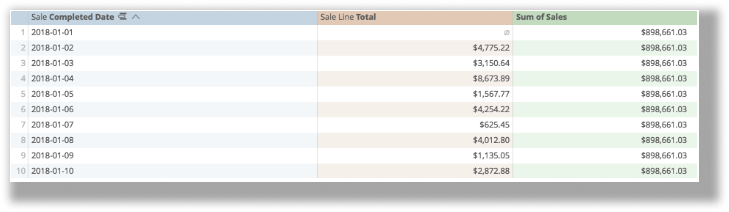# Math functions

Analytics provides lots of functions that perform different mathematical, logical, and table-based calculations. You can find a more in-depth catalog of available functions on the Looker functions and operators page.

These are categorized in five different ways:

## Large

Returns the "nth" largest value from a range, where "n" is freely defined. Requires two arguments: the range and which largest number you want to view.

For example, if I want to find my highest daily sales total, I could build...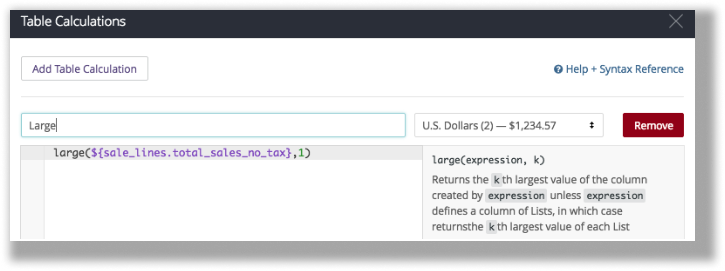The calculation:

large(\${sale_lines.total_sales_no_tax},1)

If it is saved properly: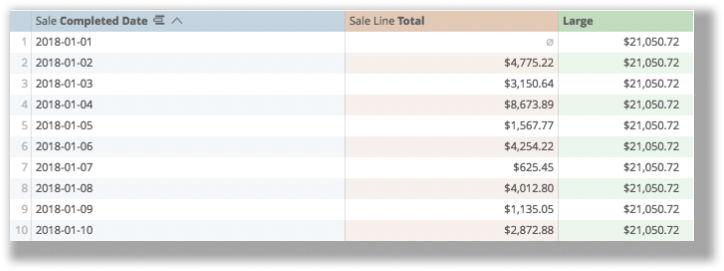Now, because the calculation looks for the first largest value, the same value appears in every row (\$21,050.72).

You can also return the row (from logic calculations) and have it provide the highest on row 1, the second-highest on row 2, the third-highest on row 3, and so on.

In this instance, the calculation looks like this:

large(\${sale_lines.total_sales_no_tax},(row()))

After saving, this is the result: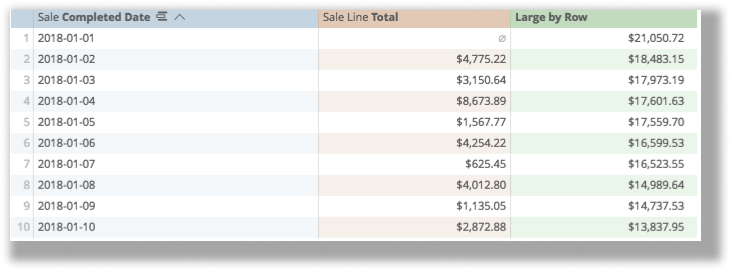## Max

Returns the largest value from a defined range. Unlike "Large", "Max" requires only one argument: defined numeric range.

## Mean, Median, Mode

Mean, Median, and Mode are the corresponding measure of average value from a range:

• Mean corresponds to the mathematical average (sum of total/count of total).
• Median corresponds to the central value of sorted results, for example in 3 results, return the 2nd, in 5 results, return the 3rd, in 101 results, return the 51st (or the average of the two central values in an even number of results).
• Mode is the most common of results.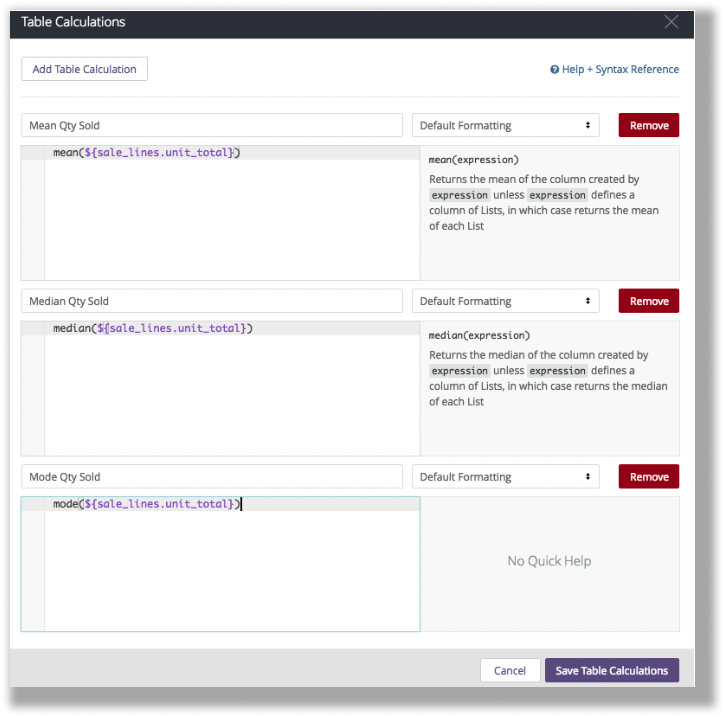This example is finding the mean, median, and mode of the number of items sold on a sale last week.

My calculations (respectively) look like this:

mean(\${sale_lines.unit_total})

median(\${sale_lines.unit_total})

mode(\${sale_lines.unit_total})

If the calculation is properly: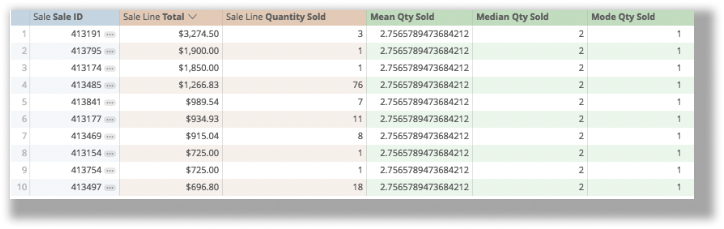## Min

Min returns the smallest value from a defined range. The required one argument: defined numeric range.

## Round

Round rounds a number up or down to a defined number of decimal places.

Say you want to look at total sales for the past six weeks and determine what the average weekly quantity sold is.

Before rounding, the weekly average looks like this: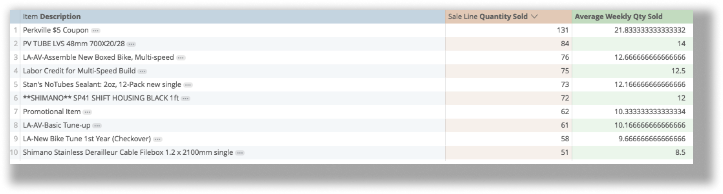The Round function needs two arguments. The first argument is which number is being rounded, and the second argument is how many decimal places we want to round to: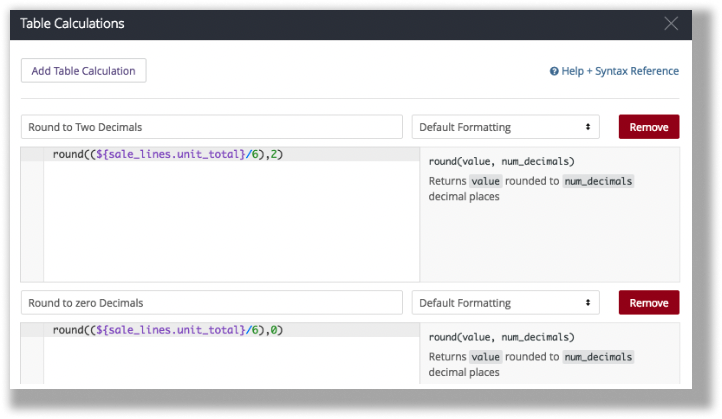In this example, the number to round is the six-week weekly average: (\${sale_lines.unit_total}/6), and the number of decimals is 2 in the first cell, and 0 in the second.

The calculations look (respectively) like this:

round((\${sale_lines.unit_total}/6),2)

round((\${sale_lines.unit_total}/6),0)

If the calculations are saved properly: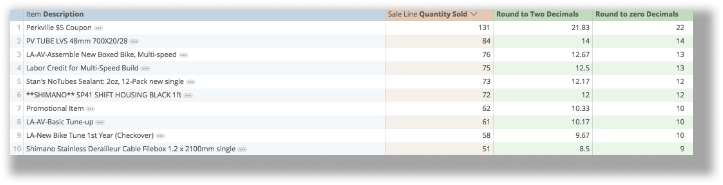## Running_total

Running_total is used to add all values in sequence, for example, to see what year-to-date totals are per day: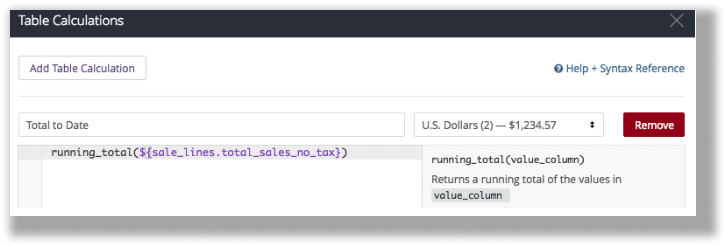In this instance, the calculation looks like this:

running_total(\${sale_lines.total_sales_no_tax})

If the calculation is saved properly: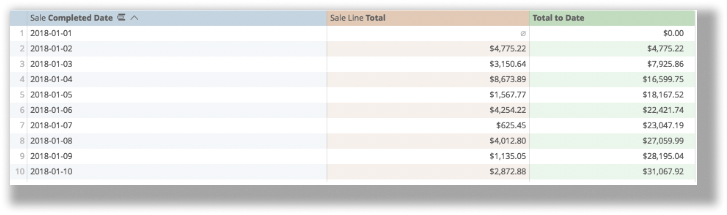## Small

Small, the opposite of Large, returns the nth smallest value from a range where n is freely defined. It requires two arguments, which field to look at, and which is the smallest number.

For example, to see what my lowest daily sales: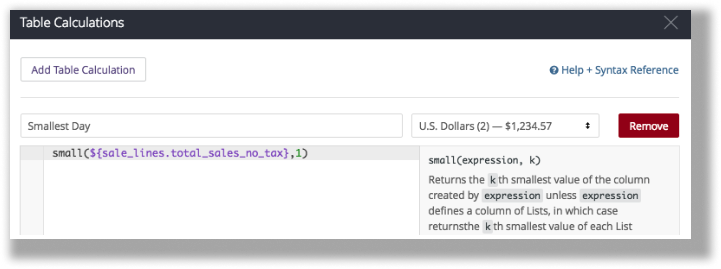The calculation looks like this:

small(\${sale_lines.total_sales_no_tax},1)

If the calculation is saved properly: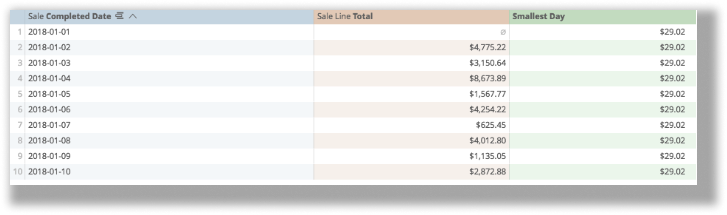## Sum

Sum returns the total value of a visible range and does not include values not displayed on the table. It requires one argument: which range is being summed.

To return the sum of total sales, for example: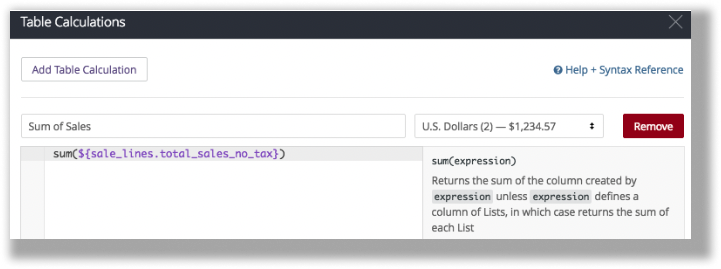Here, the calculation looks like this:

sum(\${sale_lines.total_sales_no_tax})

If saved properly, here is the result: